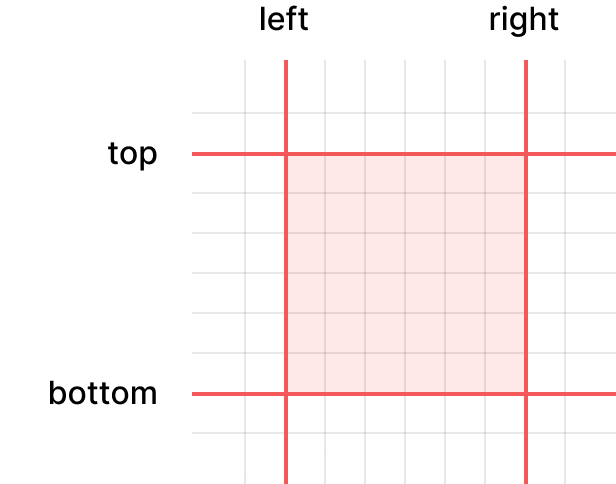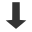Good afternoon! Let's write a toy UI library.

We begin by writing a few helper functions that will invariably prove useful to us later. We will be dealing with a lot of rectangular regions on the screen, so it makes sense to start by defining a rectangle structure and write some code to manipulate it.

``````typedef struct Rectangle {
int l, r, t, b;
} Rectangle;``````

My preferred method of encoding rectangles is as a quartet comprising the left, right, top and bottom sides.Here are the helper functions we'll implement that deal with rectangles.

``````Rectangle RectangleMake(int l, int r, int t, int b); // Initialise a Rectangle structure with the provided values.
bool RectangleValid(Rectangle a); // Returns true if the rectangle is 'valid', which I define to mean it has positive width and height.
Rectangle RectangleIntersection(Rectangle a, Rectangle b); // Compute the intersection of the rectangles, i.e. the biggest rectangle that fits into both. If the rectangles don't overlap, an invalid rectangle is returned (as per RectangleValid).
Rectangle RectangleBounding(Rectangle a, Rectangle b); // Compute the smallest rectangle containing both of the input rectangles.
bool RectangleEquals(Rectangle a, Rectangle b); // Returns true if all sides are equal.
bool RectangleContains(Rectangle a, int x, int y); // Returns true if the pixel with its top-left at the given coordinate is contained inside the rectangle.``````

If you're following along with the tutorial, I recommend trying to implement these yourself, and then comparing with my implementation. Here's a few to get you started.

``````Rectangle RectangleIntersection(Rectangle a, Rectangle b) {
if (a.l < b.l) a.l = b.l;
if (a.t < b.t) a.t = b.t;
if (a.r > b.r) a.r = b.r;
if (a.b > b.b) a.b = b.b;
return a;
}

bool RectangleContains(Rectangle a, int x, int y) {
// (x, y) gives the top-left corner of the pixel.
// Therefore we use strict inequalities when comparing against the right and bottom sides of the rectangle.
return a.l <= x && a.r > x && a.t <= y && a.b > y;
}``````

We need just one more helper function, `StringCopy`. This copies a string to a heap allocated buffer, and stores the pointer to the buffer in the given output, making sure not to leak the old buffer. I think the code is fairly self-explanatory.

``````void StringCopy(char **destination, size_t *destinationBytes, const char *source, ptrdiff_t sourceBytes) {
if (sourceBytes == -1) sourceBytes = strlen(source);
*destination = (char *) realloc(*destination, sourceBytes);
*destinationBytes = sourceBytes;
memcpy(*destination, source, sourceBytes);
}``````

While we're at it, I'll also create the one global variable we'll need for the UI library.

``````typedef struct GlobalState {
} GlobalState;

GlobalState global;``````

We'll keep all our global state together in this structure.

Here's the full code for this article. I've also added some code to check that the helper functions are doing what I want them to do. Don't forget to build with `-fsanitize=address` :)part1.c

Part 1: You are here!

Part 2: Windows.

Part 3: Elements.

Part 4: Messaging.

Part 5: Layouting.

Part 6: Painting.

Part 7: Text and boxes.

Part 8: Mouse movement.

Part 9: Mouse buttons.

Part 10: Buttons and labels.

Part 11: Panels.

Part 12: Destruction.

Part 13: An example use case.

Part 14: Changing state manually.

Part 15: Refactoring out population.

Part 16: Preparing to rewrite.

Part 17: The document-object model.

Part 18: Reading state to create the UI.

Part 19: Refactoring reactive elements.

Part 20: Saving data for reactive elements.

Part 21: Tracking reactive elements.

Part 22: Applying steps.

Part 23: Undo and redo.